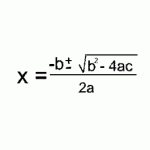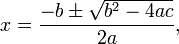Free Quadratic Equation Calculator

A quadratic equation is a polynomial equation of the second degree, generally: